## Zernike Polynomial

Orthogonal Polynomials which arise in the expansion of a wavefront function for optical systems with circular pupils. The Odd and Even Zernike polynomials are given by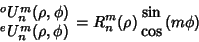(1)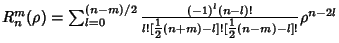(2)
forandintegers with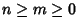and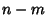Even. Otherwise,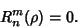(3)

Here,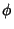is the azimuthal angle with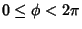and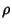is the radial distance with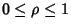(Prata and Rusch 1989). The radial functions satisfy the orthogonality relation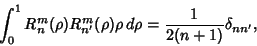(4)

where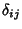is the Kronecker Delta, and are related to the Bessel Function of the First Kind by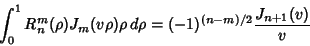(5)

(Born and Wolf 1989, p. 466). The radial Zernike polynomials have the Generating Function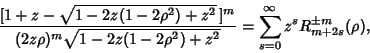(6)

and are normalized so that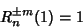(7)

(Born and Wolf 1989, p. 465). The first few Nonzero radial polynomials are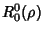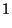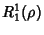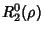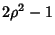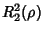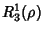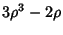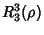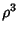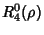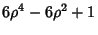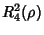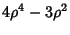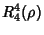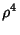(Born and Wolf 1989, p. 465).

The Zernike polynomial is a special case of the Jacobi Polynomial with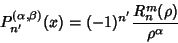(8)

and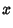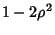(9)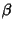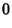(10)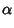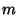(11)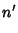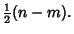(12)

The Zernike polynomials also satisfy the Recurrence Relations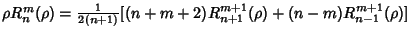(13)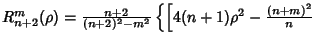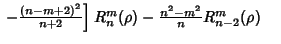(14)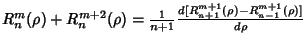(15)
(Prata and Rusch 1989). The coefficients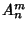and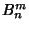in the expansion of an arbitrary radial function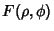in terms of Zernike polynomials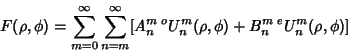(16)

are given by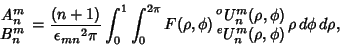(17)

where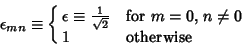(18)

Let a primary'' aberration be given by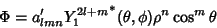(19)

with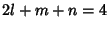and where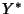is the Complex Conjugate of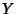, and define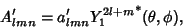(20)

giving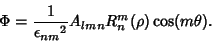(21)

Then the types of primary aberrations are given in the following table (Born and Wolf 1989, p. 470).

 Aberration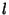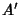spherical aberration0 4 0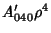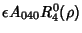coma0 3 1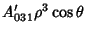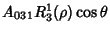astigmatism0 2 2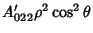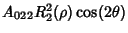field curvature1 2 0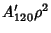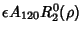distortion1 1 1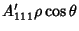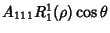References

Bezdidko, S. N. The Use of Zernike Polynomials in Optics.'' Sov. J. Opt. Techn. 41, 425, 1974.

Bhatia, A. B. and Wolf, E. On the Circle Polynomials of Zernike and Related Orthogonal Sets.'' Proc. Cambridge Phil. Soc. 50, 40, 1954.

Born, M. and Wolf, E. The Diffraction Theory of Aberrations.'' Ch. 9 in Principles of Optics: Electromagnetic Theory of Propagation, Interference, and Diffraction of Light, 6th ed. New York: Pergamon Press, pp. 459-490, 1989.

Mahajan, V. N. Zernike Circle Polynomials and Optical Aberrations of Systems with Circular Pupils.'' In Engineering and Lab. Notes 17 (Ed. R. R. Shannon), p. S-21, Aug. 1994.

Prata, A. and Rusch, W. V. T. Algorithm for Computation of Zernike Polynomials Expansion Coefficients.'' Appl. Opt. 28, 749-754, 1989.

Wang, J. Y. and Silva, D. E. Wave-Front Interpretation with Zernike Polynomials.'' Appl. Opt. 19, 1510-1518, 1980.

Zernike, F. Beugungstheorie des Schneidenverfahrens und seiner verbesserten Form, der Phasenkontrastmethode.'' Physica 1, 689-704, 1934.

Zhang, S. and Shannon, R. R. Catalog of Spot Diagrams.'' Ch. 4 in Applied Optics and Optical Engineering, Vol. 11. New York: Academic Press, p. 201, 1992.